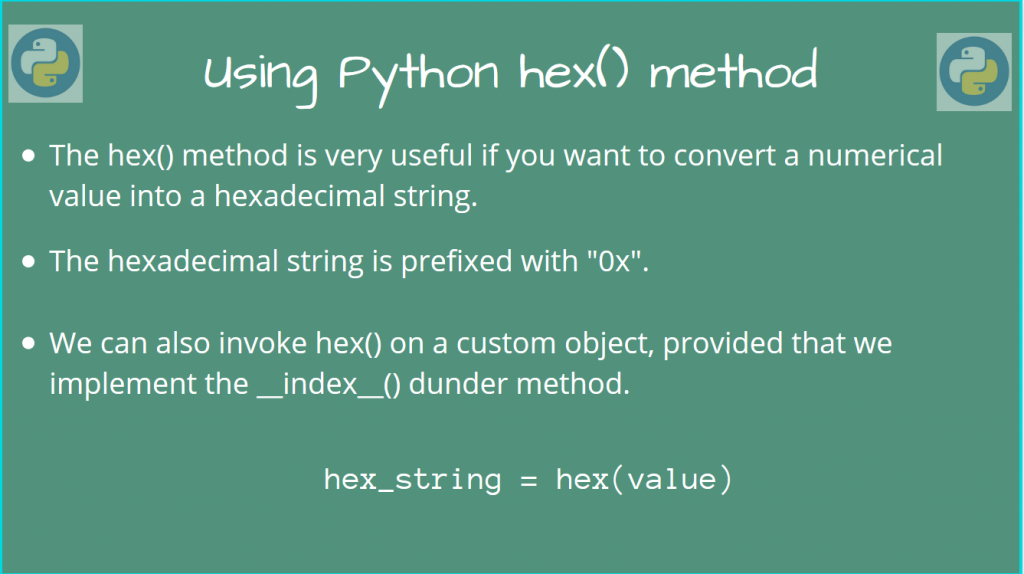# How to use the Python hex() function?This function is useful if you want to convert an Integer to a hexadecimal string, prefixed with “0x”.

Let’s look at how we could use this function.

## Using the Python hex() function

The Python hex() function has a very simple syntax:

```hex_string = hex(val)
```

Here, `val` can be an Integer, binary, octal, or a hexadecimal number.

Let’s look at some examples quickly.

```print(hex(1000))  # decimal
print(hex(0b111))  # binary
print(hex(0o77))  # octal
```

Output

```0x3e8
0x7
0x3f
0xff
```

## Using Python hex() on a custom object

We can also use hex() on a custom object. But, if we want to use it successfully, we must define the __index__() dunder method for our class.

The hex() method will call `__index__()`, so we must implement it. This must return a value, which can be a decimal / binary / octal / hexadecimal number.

```class MyClass:
def __init__(self, value):
self.value = value
def __index__(self):
print('__index__() dunder method called')
return self.value

my_obj = MyClass(255)

print(hex(my_obj))
```

Output

```__index__() dunder method called
0xff
```

Indeed, as you can see, it returns what we expected.

First, `hex()` calls the __index__ method on our custom class.

Then, it converts the returned value to a hexadecimal string (255 -> “0xff”)